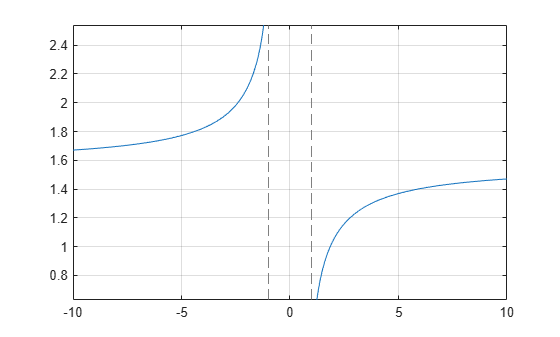# asec

Symbolic inverse secant function

## Syntax

``asec(X)``

## Description

example

````asec(X)` returns the inverse secant function (arcsecant function) of `X`. All angles are in radians. For real elements of `X` in the interval `[-Inf,-1]` and `[1,Inf]`, `asec` returns values in the interval `[0,pi]`. For real values of `X` in the interval `[-1,1]` and for complex values of `X`, `asec` returns complex values with the real parts in the interval `[0,pi]`. ```

## Examples

### Inverse Secant Function for Numeric and Symbolic Arguments

Depending on its arguments, `asec` returns floating-point or exact symbolic results.

Compute the inverse secant function for these numbers. Because these numbers are not symbolic objects, `asec` returns floating-point results.

`A = asec([-2, 0, 2/sqrt(3), 1/2, 1, 5])`
```A = 2.0944 + 0.0000i 0.0000 + Infi 0.5236 + 0.0000i... 0.0000 + 1.3170i 0.0000 + 0.0000i 1.3694 + 0.0000i```

Compute the inverse secant function for the numbers converted to symbolic objects. For many symbolic (exact) numbers, `asec` returns unresolved symbolic calls.

`symA = asec(sym([-2, 0, 2/sqrt(3), 1/2, 1, 5]))`
```symA = [ (2*pi)/3, Inf, pi/6, acos(2), 0, acos(1/5)]```

Use `vpa` to approximate symbolic results with floating-point numbers:

`vpa(symA)`
```ans = [ 2.0943951023931954923084289221863,... Inf,... 0.52359877559829887307710723054658,... 1.3169578969248165734029498707969i,... 0,... 1.3694384060045659001758622252964]```

### Plot Inverse Secant Function

Plot the inverse secant function on the interval from -10 to 10.

```syms x fplot(asec(x),[-10 10]) grid on```### Handle Expressions Containing Inverse Secant Function

Many functions, such as `diff`, `int`, `taylor`, and `rewrite`, can handle expressions containing `asec`.

Find the first and second derivatives of the inverse secant function:

```syms x diff(asec(x), x) diff(asec(x), x, x)```
```ans = 1/(x^2*(1 - 1/x^2)^(1/2)) ans = - 2/(x^3*(1 - 1/x^2)^(1/2)) - 1/(x^5*(1 - 1/x^2)^(3/2)) ```

Find the indefinite integral of the inverse secant function:

`int(asec(x), x)`
```ans = x*acos(1/x) - log(x + (x^2 - 1)^(1/2))*sign(x)```

Find the Taylor series expansion of `asec(x)` around ```x = Inf```:

`taylor(asec(x), x, Inf)`
```ans = pi/2 - 1/x - 1/(6*x^3) - 3/(40*x^5)```

Rewrite the inverse secant function in terms of the natural logarithm:

`rewrite(asec(x), 'log')`
```ans = -log(1/x + (1 - 1/x^2)^(1/2)*1i)*1i```

## Input Arguments

collapse all

Input, specified as a symbolic number, variable, expression, or function, or as a vector or matrix of symbolic numbers, variables, expressions, or functions.

## Version History

Introduced before R2006a